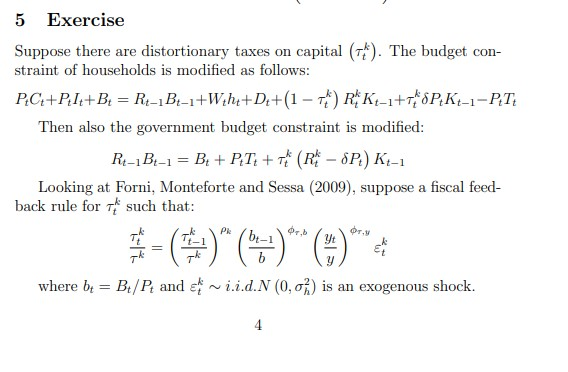# Transcription of the equation on dynare

Good evening,

I’m having a problem transcribing the government budget constraint equation (on page 4 of the file) into dynare.

Gruppo14.pdf (134.5 KB)

How would you write the government budget constraint?

For example this is how the budget constraint is written, about file Gruppo15:

% 13 Government budget constraint

But about file Gruppo 14, how can I write the Government budget constraint?
Gruppo14.pdf (134.5 KB)
Gruppo15.pdf (135.0 KB)

Thank you so much for the courtesy.
The error connected with the project (correlated with the prior pdf) are the follower:

1. For Dynare: Symbol tauk is being treated as if it were a function (i.e., takes an argument that is not an integer).
2. We don’t know how to elaborate the government budget constraint represented in the page 4 of file (point 1 of the exercise, where there is the budget constraint modified
3. We don’t know if we are missing equations (like government spending)
provafinal.mod (2.9 KB)
1. You forgot a multiplication sign. provafinal.mod (2.9 KB)
2. What is the question? You are given the equation and you should be able to directly implement it in Dynare.
3. It seems you are missing the household budget constraint.

The question is connected with this exercise: how it is possible to write the budget constraint following the second equation?
For the household budget constraint we are checking now if it is correct. (I really appreciate your work, so sorry for all this question)But the equation is right there. Or is the problem that you are working with a linearized model?

We are working on log linearized model and the main challenge is how to write the government budget constraint and the household budget constraint (not using B or P in the model, the 13)equation in the file mod is connected with a similar exercise)

Why don’t you simply code the nonlinear equations. One problem with linearization is that the absolute price level is typically indeterminate. That would require you to deflate the budget constraint.